# The Hosmer-Lemeshow goodness of fit test for logistic regression

Before a model is relied upon to draw conclusions or predict future outcomes, we should check, as far as possible, that the model we have assumed is correctly specified. That is, that the data do not conflict with assumptions made by the model. For binary outcomes logistic regression is the most popular modelling approach. In this post we’ll look at the popular, but sometimes criticized, Hosmer-Lemeshow goodness of fit test for logistic regression.

The logistic regression model
We will assume we have binary outcome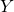and covariates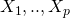. The logistic regression model assumes that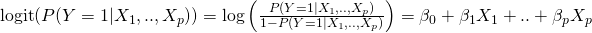This implies that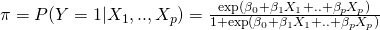The unknown model parameters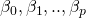are ordinarily estimated by maximum likelihood. In R this is performed by the glm (generalized linear model) function, which is part of the core stats library. We will write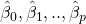for the maximum likelihood estimates of the parameters.

The Hosmer-Lemeshow goodness of fit test
The Hosmer-Lemeshow goodness of fit test is based on dividing the sample up according to their predicted probabilities, or risks. Specifically, based on the estimated parameter values, for each observation in the sample the probability that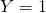is calculated, based on each observation’s covariate values: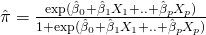The observations in the sample are then split into g groups (we come back to choice of g later) according to their predicted probabilities. Suppose (as is commonly done) that g=10. Then the first group consists of the observations with the lowest 10% predicted probabilities. The second group consists of the 10% of the sample whose predicted probabilities are next smallest, etc etc.

Suppose for the moment, artifically, that all of the observations in the first group had a predicted probability of 0.1. Then, if our model is correctly specified, we would expect the proportion of these observations who haveto be 10%. Of course, even if the model is correctly specified, the observed proportion will deviate to some extent from 10%, but not by too much. If the proportion of observations within the group were instead 90%, this is suggestive that our model is not accurately predicting probability (risk), i.e. an indication that our model is not fitting the data well.

In practice, as soon as some of our model covariates are continuous, each observation will have a different predicted probability, and so the predicted probabilities will vary in each of the groups we have formed. To calculate how manyobservations we would expect, the Hosmer-Lemeshow test takes the average of the predicted probabilities in the group, and multiplies this by the number of observations in the group. The test also performs the same calculation for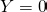, and then calculates a Pearson goodness of fit statistic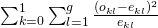where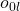denotes the number of observedobservations in theth group,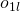denotes the number of observedobservations in theth group, and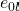and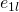similarly denote the expected number of zeros.

In a 1980 paper Hosmer-Lemeshow showed by simulation that (provided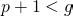) their test statistic approximately followed a chi-squared distribution on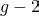degrees of freedom, when the model is correctly specified. This means that given our fitted model, the p-value can be calculated as the right hand tail probability of the corresponding chi-squared distribution using the calculated test statistic. If the p-value is small, this is indicative of poor fit.

It should be emphasized that a large p-value does not mean the model fits well, since lack of evidence against a null hypothesis is not equivalent to evidence in favour of the alternative hypothesis. In particular, if our sample size is small, a high p-value from the test may simply be a consequence of the test having lower power to detect mis-specification, rather than being indicative of good fit.

Choosing the number of groups
As far as I have seen, there is little guidance as to how to choose the number of groups g. Hosmer and Lemeshow’s conclusions from simulations were based on using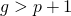, suggesting that if we have 10 covariates in the model, we should choose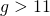, although this doesn’t appear to be mentioned in text books or software packages.

Intuitively, using a small value of g ought to give less opportunity to detect mis-specification. However, if we choose g to large, the numbers in each group may be so small that it will be difficult to determine whether differences between observed and expected are due to chance or indicative or model mis-specification.

A further problem, highlighted by many others (e.g. Paul Allison) is that, for a given dataset, if one changes g, sometimes one obtains a quite different p-value, such that with one choice of g we might conclude our model does not fit well, yet with another we conclude there is no evidence of poor fit. This is indeed a troubling aspect of the test.

Hosmer-Lemeshow in R
R’s glm function cannot perform the Hosmer-Lemeshow test, but many other R libraries have functions to perform it. Below I illustrate using the hoslem.test function in the ResourceSelection library to do this, but I’ve also put together a short YouTube video illustrating the function:

First we will simulate some data from a logistic regression model with one covariate x, and then fit the correct logistic regression model. This means our model is correctly specified, and we should hopefully not detect evidence of poor fit.

library(ResourceSelection)
set.seed(43657)
n <- 100
x <- rnorm(n)
xb <- x
pr <- exp(xb)/(1+exp(xb))
y <- 1*(runif(n) < pr)
mod <- glm(y~x, family=binomial)

Next we pass the outcome y and model fitted probabilities to the hoslem.test function, choosing g=10 groups:

hl <- hoslem.test(mod$y, fitted(mod), g=10) hl Hosmer and Lemeshow goodness of fit (GOF) test data: mod$y, fitted(mod)
X-squared = 7.4866, df = 8, p-value = 0.4851


This gives p=0.49, indicating no evidence of poor fit. This is good, since here we know the model is indeed correctly specified. We can also obtain a table of observed vs expected, from our hl object:

cbind(hl$observed,hl$expected)
y0 y1    yhat0    yhat1
[0.0868,0.219]  8  2 8.259898 1.740102
(0.219,0.287]   7  3 7.485661 2.514339
(0.287,0.329]   7  3 6.968185 3.031815
(0.329,0.421]   8  2 6.194245 3.805755
(0.421,0.469]   5  5 5.510363 4.489637
(0.469,0.528]   4  6 4.983951 5.016049
(0.528,0.589]   5  5 4.521086 5.478914
(0.589,0.644]   2  8 3.833244 6.166756
(0.644,0.713]   6  4 3.285271 6.714729
(0.713,0.913]   1  9 1.958095 8.041905

To help us understand the calculation, let's now perform the test ourselves manually. First we calculate the model predicted probabilities, and then categorise the observations according to deciles of the predicted probabilities:

pihat <- mod$fitted pihatcat <- cut(pihat, breaks=c(0,quantile(pihat, probs=seq(0.1,0.9,0.1)),1), labels=FALSE) Next, we cycle through the groups 1 to 10, counting the number observed 0s and 1s, and calculating the expected number of 0s and 1s. To calculate the latter, we find the mean of the predicted probabilities in each group, and multiply this by the group size, which here is 10: meanprobs <- array(0, dim=c(10,2)) expevents <- array(0, dim=c(10,2)) obsevents <- array(0, dim=c(10,2)) for (i in 1:10) { meanprobs[i,1] <- mean(pihat[pihatcat==i]) expevents[i,1] <- sum(pihatcat==i)*meanprobs[i,1] obsevents[i,1] <- sum(y[pihatcat==i]) meanprobs[i,2] <- mean(1-pihat[pihatcat==i]) expevents[i,2] <- sum(pihatcat==i)*meanprobs[i,2] obsevents[i,2] <- sum(1-y[pihatcat==i]) } Lastly, we can calculate the Hosmer-Lemeshow test statistic by the sum of (observed-expected)^2/expected across the 10x2 cells of the table: hosmerlemeshow <- sum((obsevents-expevents)^2 / expevents) hosmerlemeshow  7.486643  in agreement with the test statistic value from the hoslem.test function. Changing the number of groups Next, let's see how the test's p-value changes as we choose g=5, g=6, up to g=15. We can do this with a simple for loop: for (i in 5:15) { print(hoslem.test(mod$y, fitted(mod), g=i)$p.value) }  which gives  0.4683388  0.9216374  0.996425  0.9018581  0.933084  0.4851488  0.9374381  0.9717069  0.5115724  0.4085544  0.8686347  Although the p-values are changing somewhat, they are all clearly non-significant, so they are giving a similar conclusion, that there is no evidence of poor fit. So for this dataset, choosing different values of g doesn't seem to affect the substantive conclusion. Checking the Hosmer-Lemeshow test through simulation To finish, let's perform a little simulation to check how well the Hosmer-Lemeshow test performs in repeated samples. First, we will repeatedly sample from the same model as used previously, fit the same (correct) model, and calculate the Hosmer-Lemeshow p-value using g=10. We will do this 1,000 times, and store the test p-values in an array: pvalues <- array(0, 1000) for (i in 1:1000) { n <- 100 x <- rnorm(n) xb <- x pr <- exp(xb)/(1+exp(xb)) y <- 1*(runif(n) < pr) mod <- glm(y~x, family=binomial) pvalues[i] <- hoslem.test(mod$y, fitted(mod), g=10)$p.value } When completed, we can calculate the proportion of p-values which are less than 0.05. Since the model is correctly specified here, we want this so called type 1 error rate to be no larger than 5%: mean(pvalues<=0.05)  0.04  So, from 1,000 simulations, the Hosmer-Lemeshow test gave a significant p-value, indicating poor fit, on 4% of occasions. So the test is wrongly suggesting poor fit within the 5% limit we would expect - it seems to be working ok. Now let's change the simulation so that the model we fit is incorrectly specified, and should fit the data poorly. Hopefully we will find that the Hosmer-Lemeshow test correctly finds evidence of poor fit more often than 5% of the time. Specifically, we will now generateto follow a logistic model with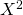as covariate, but we will continue to fit the model with linear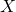as covariate, such that our fitted model is incorrectly specified. To do this we just change the line which generates the linear predictor as being equal to: for (i in 1:1000) { n <- 100 x <- rnorm(n) xb <- x^2 pr <- exp(xb)/(1+exp(xb)) y <- 1*(runif(n) < pr) mod <- glm(y~x, family=binomial) pvalues[i] <- hoslem.test(mod$y, fitted(mod), g=10)$p.value }  Calculating the proportion of p-values less than 0.05 we find mean(pvalues<=0.05)  0.648  So, the Hosmer-Lemeshow test gives us significant evidence of poor fit on 65% of occasions. That it does not detect the poor fit more often is at least partly a consequence of power - with a larger sample size the power to detect poor fit (or indeed anything!) will be larger. Of course this is just a very simple simulation study, but it is nice to see that, at least for the setup we have used, the test performs as we would hope. Lastly, a comment. One limitation of 'global' goodness of fit tests like Hosmer-Lemeshow is that if one obtains a significant p-value, indicating poor fit, the test gives no indication as to in what respect(s) the model is fitting poorly. Books For more detailed information on the Hosmer-Lemeshow test, and its justification, I'd recommend looking at Hosmer and Lemeshow's (and now also Sturdivant) book, Applied Logistic Regression. For more general reading on approaches for assessing logistic (and other) regression models, both in terms of goodness of fit (calibration), and predictive ability (discrimination), I'd recommend looking at Harrell's Regression Modelling Strategies book, or Steyerberg's Clinical Prediction Models book. ### 33 thoughts on “The Hosmer-Lemeshow goodness of fit test for logistic regression” 1.Dear Jonathan, As far as I know, the Hosmer-Lemeshow goodness of fit test is overall used to assess goodness of fit in logistic regression with individual binary data. Would it be sensible to use it also for grouped binary data? I understand that, in general, grouped binary data implies that some (or a few – at least not an infinite number of) groups of individuals share the same probability of success. Then, it seems reasonable to use the Deviance as a measure of goodness or badness of fit. However, what happens if the number of such groups which share the same probability (and for which p=r/n) is very large (say >100)? Would it still be reasonable to use the Deviance as ‘badness’ of fit? Or would it be more sensible to use the Hosmer-Lemeshow test? Thank you very much… Reply •Hi Carmen As you say, in the case of grouped binomial data, the deviance can usually be used to assess whether there is evidence of poor fit. The deviance test is a likelihood ratio test comparing the current model to the saturated model, but it shouldn’t be used with individual binary data. You mention a situation where the data are grouped binomial, but there are a large number of groups. In this situation, I believe the deviance goodness of fit test should be fine, provided the n’s in the groups are reasonably large. As to how Hosmer-Lemeshow would perform in this situation, to be honest I’m not sure. Best wishes Jonathan Reply •Hi Johnathan, May I know why deviance test should not be use for individual binary data. Reply •The deviance test is not valid for individual binary data because the deviance test is essentially a log likelihood ratio test comparing your model to the saturated model. With individual binary data the number of parameters in the saturated model grows at the same rate as the sample size, which violates an assumption needed for the asymptotic validity of the likelihood ratio test. Reply 2.Thank you so much! Best wishes, Carmen Reply 3.Of course, even if the model is correctly specified, the observed proportion will deviate to some extent from 10%, but not by too much. The word ‘correctly’ should be replaced with ‘incorrectly’. Reply •Thanks for your comment Anvesh, but I don’t understand why you think “correctly” should be changed to “incorrectly” here. If the model is not correctly specified, in general the model won’t have good calibration and so we will get systematic differences between observed and predicted. All I was saying here is that even if the model is correctly specified, the observed and expected proportions will not be the same exactly due to sampling variation and also because the predictions are made based on estimated parameter values rather than the true parameter values. Reply 4.Thanks for the illustration for my logistic regression model i have the dependent variable as a matrix cbind(k, n-k) which gives the number of success and number of failures . i do have many independent variables, some categorical, some continous. in this case how should hoslem.test be performed in R. in particular : hl <- hoslem.test(mod$y, fitted(mod), g=10) ; i have the above matrix instead of binary y in the example given.

•Hi. If you’ve fitted the model using the GLM function, the fitted model object (what I called mod in the R code in the post) contains the vector y of binary outcomes. So you should be able to take your fitted model object (mod, or whatever you’ve called it), and then apply the Hosmer-Lemeshow test using the same code, i.e. hl <- hoslem.test(mod$y, fitted(mod), g=10) Reply •Hi for some modelling approaches like random survival forest, we dont get binary output. How can we apply hoslem test for those continuous cases? Thank you Reply •The Hosmer-Lemeshow test is designed to assess goodness of fit in the case of binary outcomes. Various different approaches have been developed for assessing goodness of fit for survival models, but I’m not so familiar with these I’m afraid. Reply 5.You state: “It should be emphasized that a large p-value does not mean the model fits well, since lack of evidence against a null hypothesis is not equivalent to evidence in favour of the alternative hypothesis. In particular, if our sample size is small, a high p-value from the test may simply be a consequence of the test having lower power to detect mis-specification, rather than being indicative of good fit.” Just want to clarify: isn’t the null hypothesis of the Hosmer-Lemeshow goodness of fit test that there is a “non-poor” fit, and the alternative (P< 0.05) is a poor fit? If it is, doesn't your statement above need to reverse the "null" and "alternative" words so that the paragraph becomes: "It should be emphasized that a large p-value does not mean the model fits well, since lack of evidence against an alternative hypothesis is not equivalent to evidence in favour of the null hypothesis. In particular, if our sample size is small, a high p-value from the test may simply be a consequence of the test having lower power to detect mis-specification, rather than being indicative of good fit." Thanks for any clarifications. Reply •Thanks, but I think it is correct as written: the null hypothesis of the test is that the model is correctly specified. If we have p<0.05, we have evidence to reject this null hypothesis, meaning we have evidence that the model is not correctly specified, and doesn't fit the data well. Conversely, a non-significant Hosmer-Lemeshow test result, while consistent with the null hypothesis that our model is correctly specified / fits the data well, it doesn't prove it. Reply 6.Hi Jonathan, Thanks for your Youtube video on how to do the Hosmer-Lemeshow test in R. I was hoping the process would be as straightforward for my dataset, but when I attempt to run the “hoslem.test” function on the model, I am getting the following error message: “Error in model.frame.default(formula = cbind(y0 = 1 – y, y1 = y) ~ cutyhat) : variable lengths differ (found for ‘cutyhat’)”. Some info about my test: I am trying to run the H-L test for my global logistic model, which contains three 2-way interactions between categorical variables and a single continuous main effect. I have 332 observations with a binary response variable (“1″ for presence/”0” for absence). Do you know what this error message means and how I might address it so that I may run the test? There are different numbers of observations for different levels of categorical variables. Could this be the problem? If so, is there anything I can do about that without getting rid of data? Regards, Robin Reply •Hi Robin I’m not sure what the error means I’m afraid. But I’m also not sure what you mean by “there are different numbers of observations for the different levels of categorical variables” – do you mean you have missing values in some of the predictors (which may cause an issue)? Or do you mean the number of levels is different for the different categorical variables (which should be fine). Best wishes Jonathan Reply •Hey Robin, I’m getting the same error. Have you figured a way around it? Thanks, Christy Reply 7.Hi Jonathan, You say: “In a 1980 paper Hosmer-Lemeshow showed by simulation that (provided p+1<g ) their test statistic approximately followed a chi-squared distribution on g−2 degrees of freedom…" If this is true, I find it shocking – I don't have the original paper, but I have not seen this constraint mentioned anywhere – neither in documentation, or discussion sites. If it's in their book, I missed it. I have seen this test applied routinely in situations which violate this constraint… Can you perhaps quote the relevant excerpt from the paper? I am running into skepticism, since this seems to be so little known… Thanks, Michael Reply •Thanks Michael. I don’t have the 1980 paper, but I have the 1982 paper by Lemeshow and Hosmer ‘A review of goodness of fit statistics for use in the development of logistic regression models’, American Journal of Epidemiology 115:92-106. In this they write (page 96) in reference to their statistic that: The theoretical development given by Hosmer and Lemeshow (1980) requires only that g>(p+1). and following this that Hosmer and Lemeshow (1980) have shown via computer simulations that if the number of covariates plus one is less than the number of groups (i.e. p+1 < g), then the statistic C*_g has a distribution which is closely approximated by a chi-square distribution with g-2 degrees of freedom when H_0 is true. I too have not seen this condition mentioned in their (or others) books, and indeed was only told about it by a student who had found it in this paper. Reply 8.Hi Jonathan, thank you for the very interesting post. I think a part of your code is slightly incorrect. Namely, instead of: expevents[i,2] <- sum(pihatcat==i)*meanprobs[i,2]*meanprobs[i,2] there should be: expevents[i,2] <- sum(pihatcat==i)*meanprobs[i,2] (I guess this is just a copy&paste type of error). Could you please clarify this issue? Thanks again., Wojciech Reply •Many thanks Wojciech! I don’t know how that crept in there! I have corrected it now. Reply 9.Hi Jonathan, I have a question when it comes to “changing the number of groups”. You first select a g=10, but HS said that p+1 < g. I know there seems to be no reason number of groups to select, but then you vary the group size from 5:15. Why did you choose such a width? Did you consider your sample size? But overal, thanks for a great explaination and showing other methods one can simulate to asses the hypothesis. Warm regards, Luke Reply •Hi Luke Thanks! I just picked 5 to 15 fairly arbitrarily, as values around the usual default of 10. Best wishes Jonathan Reply 10.Hi Jonathan, I am getting non-significant HL results from my large dataset of 68000 cases. However, when I take a 10% random sample of the dataset, HL becomes significant. What do you recommend? Reply •Wow – with such a large dataset you should have very good power to detect any model misspecification, so having a non-significant result is very surprising. Moreover, I can’t see any particular reason that the HL test should give you a significant result when you fit the model to a random subset, apart from it just being a chance result (as opposed to something systematic). Reply 11.This was an absolutely fantastic tutorial. Reply 12.In the expected frequencies, some cells are less than 5, is this normal? because as I read in the book it says that the expected frequencies should not be less that 5. in addition to that, I got s sample with a dependent variable ranging from (ordered) (1 to 4). would H-L test be a valid test for this nature of data ? because when I run the analysis, it gives me a df = 23. which means there is something wrong and can not figure out what is it. Reply 13.HI Jonathan- excellent page and very helpful video. I’m trying to learn R by copying code as you have presented. I have my own data set and a model. When I use this code hl <- hoslem.test(mod$y, fitted(mod), g=10) I put the name of my model in place of 'mod' but I'm not sure what I should put in place of the 'y'? Any advice gratefully received.
Regards
Tom

14.Hi Jonathan,

Very interesting read. Thanks so much for your patience in answering all queries. I am coming across a very peculiar issue. the last two log regression models that I ran registered high AUC values but failed the HL test. I could understand if this happened on a one-off model but this seems to be a trend. Where could I be going wrong? The first test used 1497 observations and the second ( a very simple glm using just 3 variables) used 400 observations. I am really new to this and I don’t really understand the complicated math behind this, but am wondering how to interpret the results.

•Hi Sujatha. The AUC is high if the model is good at discriminating between high risk and low risk individuals. But it doesn’t assess whether the predicted risks match the observed risks, which is calibration. The HL test is assessing calibration – the model could be poorly calibrated but still have good discrimination.

•Thanks Jon, I re-ran the model using different train-test datasets and found that at times the model had a good / acceptable HL score. What can we infer from that? Also, could you direct me to some studies that help us calibrate our models? What techniques are normally used?

15.Hi Jonathan! I’m not a mathematician myself but I got to deal with the stats texts as a translator. I’ve come across this sentence: ‘The calculation of this statistics [Hosmer-Lemeshow] is the best way to verify the quality of the binary logistic regression model built in SPSS’. And I’m not sure if it’s factually correct. Is it really the best way or just the best way that our statistician is aware of. Could you please help me with this?

••